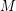# Which homology 3-spheres bound homology 4-balls?

 Importance: Outstanding ✭✭✭✭
 Author(s): Ancient/folklore
 Subject: Topology
 Keywords: cobordism homology ball homology sphere
 Posted by: rybu on: November 7th, 2009
Problem   Is there a complete and computable set of invariants that can determine which (rational) homology-spheres bound (rational) homology-balls?

Determining which homology-spheres bound homology-balls is a long standing open problem in 3/4-manifold topology. Much effort has gone towards understanding the situation for the Brieskorn homology spheres. For example, the Poincare Dodecahedral space is known not to bound a homology-ball since the Rochlin invariant is non-trivial -- butthe connect-sum of Poincare Dodecahedral spacewith its orientation-reverse does bound a homology 4-ball, and it has a simple construction: remove an open tubular neighbourhood offrom, this is the-manifold.

Standard invariants used to show homology-spheres do not bound homology-balls are various spin or spin^c cobordism invariants such as: the Rochlin invariant, Siebenmann's-invariant, the Oszvath-Szabo-invariant, and there are many others.

## Bibliography

[K] Kirby, Robion (1989), The topology of 4-manifolds, Lecture Notes in Mathematics, 1374, Springer-Verlag,

[R] Rokhlin, Vladimir A, New results in the theory of four-dimensional manifolds, Doklady Acad. Nauk. SSSR (N.S.) 84 (1952) 221-224.

[AK] S.Akbulut, R.Kirby, "Mazur manifolds," Michigan Math. J. 26 (1979), 259--284.

[CH] A.Casson, J.Harer, "Some homology lens spaces which bound rational homology balls." Pacific. J. Math. Vol 96, No 1, (1981) 23–36.

[F] H.Fickle, "Knots, Z-Homology 3-spheres and contractible 4-manifolds," pp. 467--493, Houston J. Math. Vol 10, No. 4 (1984).

[FS] R.Fintushel, R.Stern, "An exotic free involution on S^4," Ann. Math. (2) 113 (1981) no2, 357--365.

[M] B.Mazur, "A note on some contractible 4-manifolds", Annals of Mathematics, (2) 73 (1961). 221–228.

[S] R.Stern,"Some Brieskorn spheres which bound contractible manifolds," Notices Amer. Math. Soc 25 (1978), A448.

[L] Lisca, Paolo Sums of lens spaces bounding rational balls. Algebr. Geom. Topol. 7 (2007), 2141--2164.

* indicates original appearance(s) of problem.